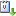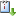Usted está aquí: On a quasilinear Schrödinger equation

# On a quasilinear Schrödinger equation

Andrzej Szulkin (Stockholm University), Jueves 26 de septiembre de 2013, 11:00 hrs.
Ponente:
Cuándo 26/09/2013 de 11:00 a 12:00 Graciela SalicrupvCaliCal

We consider the semilinear Schrödinger equation $-\Delta u+V(x)u-\Delta(u^{2})u=g(x,u), \quad x\in \mathbb{R}^{N},$ where $$g$$ and $$V$$ are periodic in $$x_1,\ldots,x_N, V>0, g$$ is odd in $$u$$ and of subcritical growth in the sense that $$|g(x,u)|\leq a(1+|u|^{p-1})$$, where $$4<p<2\cdot 2^*$$. We show that this equation has infinitely many geometrically distinct solutions in each of the following two cases:

(i)  $$g(x,u)=o(u)$$ as $$u\to 0$$, $$G(x,u)/u^4\to\infty$$ as $$|u|\to\infty$$, where $$G$$ is the primitive of $$g$$, and $$u\mapsto g(x,u)/u^3$$ is positive for $$u\ne 0$$, nonincreasing on $$(-\infty, 0)$$ and nondecreasing on $$(0, \infty)$$.

(ii) $$g(x,u)=q(x)u^3$$, where $$q>0$$.

The argument uses the Nehari manifold technique. A special feature here is that the Nehari manifold is not likely to be of class $$C^1$$.

This is joint work with Xiangdong Fang.